# Charting

## Seaborn

### Introduction

seaborn is a data visualization library based on matplotlib. It makes it easier to create more complicated plots and allows us to create much more visually-appealing charts than matplotlib charts.

### Import Libraries

Follow these steps to import the libraries that you need:

1. Import the seaborn and matplotlib libraries.
2. import seaborn as sns
import matplotlib.pyplot as plt
3. Import, and then call, the register_matplotlib_converters method.
4. from pandas.plotting import register_matplotlib_converters
register_matplotlib_converters()

### Get Historical Data

Get some historical market data to produce the plots. For example, to get data for a bank sector ETF and some banking companies over 2021, run:

qb = QuantBook()
tickers = ["XLF",   # Financial Select Sector SPDR Fund
"COF",   # Capital One Financial Corporation
"GS",    # Goldman Sachs Group, Inc.
"JPM",   # J P Morgan Chase & Co
"WFC"]   # Wells Fargo & Company
symbols = [qb.AddEquity(ticker, Resolution.Daily).Symbol for ticker in tickers]
history = qb.History(symbols, datetime(2021, 1, 1), datetime(2022, 1, 1))

### Create Candlestick Chart

Seaborn does not currently support candlestick charts. Use one of the other plotting libraries to create candlestick charts.

### Create Line Chart

You must import the plotting libraries and get some historical data to create line charts.

In this example, you create a line chart that shows the closing price for one of the banking securities. Follow these steps to create the chart:

1. Select a Symbol.
2. symbol = symbols
3. Slice the history DataFrame with the symbol and then select the close column.
4. data = history.loc[symbol]['close']
5. Call the DataFrame constructor with the data Series and then call the reset_index method.
6. data = pd.DataFrame(data).reset_index()
7. Call the lineplot method with the data Series and the column name of each axis.
8. plot = sns.lineplot(data=data,
x='time',
y='close')
9. In the same cell that you called the lineplot method, call the set method with the y-axis label and a title.
10. plot.set(ylabel="price", title=f"{symbol} Price Over Time");

The Jupyter Notebook displays the line chart.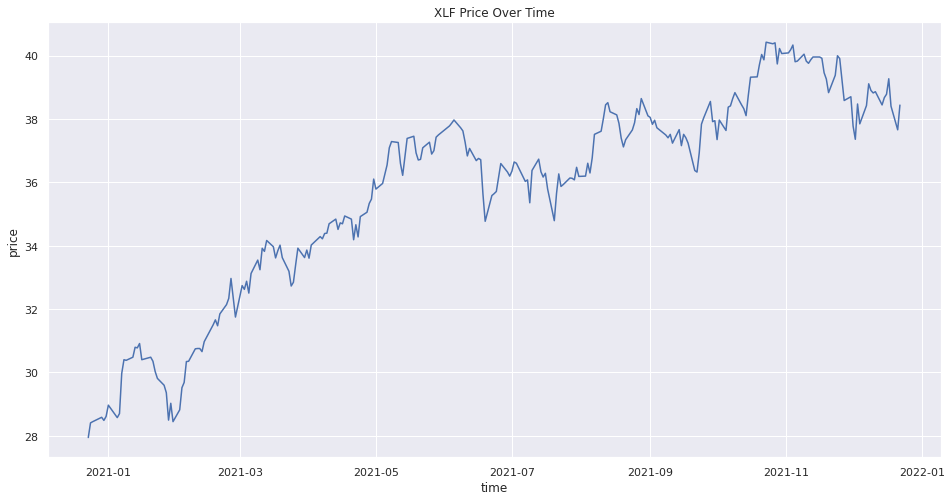### Create Scatter Plot

You must import the plotting libraries and get some historical data to create scatter plots.

In this example, you create a scatter plot that shows the relationship between the daily returns of two banking securities. Follow these steps to create the scatter plot:

1. Select 2 Symbols.
2. For example, to select the Symbols of the first 2 bank stocks, run:

symbol1 = symbols
symbol2 = symbols
3. Select the close column of the history DataFrame, call the unstack method, and then select the symbol1 and symbol2 columns.
4. close_prices = history['close'].unstack(0)[[symbol1, symbol2]]
5. Call the pct_change method and then call the dropna method.
6. daily_returns = close_prices.pct_change().dropna()
7. Call the regplot method with the daily_returns DataFrame and the column names.
8. plot = sns.regplot(data=daily_returns,
x=daily_returns.columns,
y=daily_returns.columns)
9. In the same cell that you called the regplot method, call the set method with the axis labels and a title.
10. plot.set(xlabel=f'{daily_returns.columns} % Returns',
ylabel=f'{daily_returns.columns} % Returns',
title=f'{symbol1} vs {symbol2} Daily % Returns');

The Jupyter Notebook displays the scatter plot.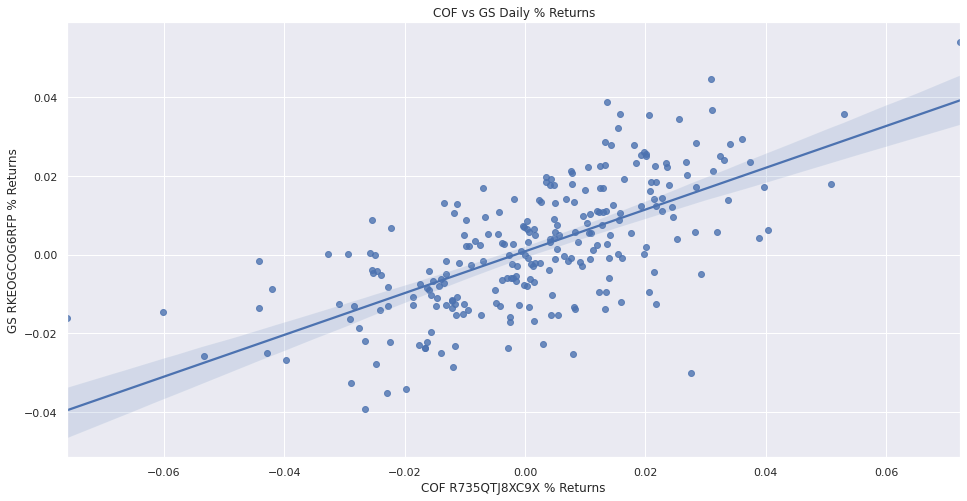### Create Histogram

You must import the plotting libraries and get some historical data to create histograms.

In this example, you create a histogram that shows the distribution of the daily percent returns of the bank sector ETF. Follow these steps to create the histogram:

1. Select the Symbol.
2. symbol = symbols
3. Slice the history DataFrame with the symbol and then select the close column.
4. data = history.loc[symbol]['close']
5. Call the pct_change method and then call the dropna method.
6. daily_returns = data.pct_change().dropna()
7. Call the DataFrame constructor with the daily_returns Series and then call the reset_index method.
8. daily_returns = pd.DataFrame(daily_returns).reset_index()
9. Call the histplot method with the daily_returns, the close column name, and the number of bins.
10. plot = sns.histplot(daily_returns, x='close', bins=20)
11. In the same cell that you called the histplot method, call the set method with the axis labels and a title.
12. plot.set(xlabel='Return',
ylabel='Frequency',
title=f'{symbol} Daily Return of Close Price Distribution');

The Jupyter Notebook displays the histogram.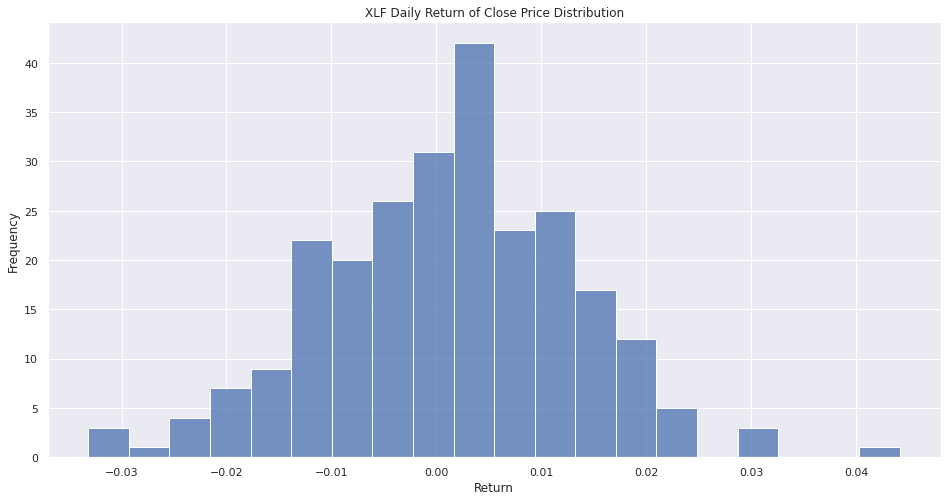### Create Bar Chart

You must import the plotting libraries and get some historical data to create bar charts.

In this example, you create a bar chart that shows the average daily percent return of the banking securities. Follow these steps to create the bar chart:

1. Select the close column and then call the unstack method.
2. close_prices = history['close'].unstack(level=0)
3. Call the pct_change method and then multiply by 100.
4. daily_returns = close_prices.pct_change() * 100
5. Call the mean method.
6. avg_daily_returns = daily_returns.mean()
7. Call the DataFrame constructor with the avg_daily_returns Series and then call the reset_index method.
8. avg_daily_returns = pd.DataFrame(avg_daily_returns, columns=["avg_daily_ret"]).reset_index()
9. Call barplot method with the avg_daily_returns Series and the axes column names.
10. plot = sns.barplot(data=avg_daily_returns, x='symbol', y='avg_daily_ret')
11. In the same cell that you called the barplot method, call the set method with the axis labels and a title.
12. plot.set(xlabel='Tickers',
ylabel='%',
title='Banking Stocks Average Daily % Returns')
13. In the same cell that you called the set method, call the tick_params method to rotate the x-axis labels.
14. plot.tick_params(axis='x', rotation=90)

The Jupyter Notebook displays the bar chart.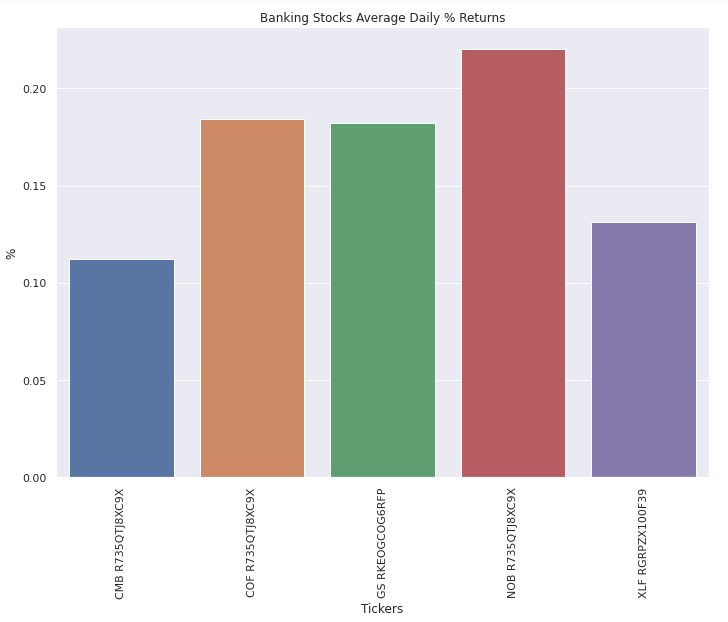### Create Heat Map

You must import the plotting libraries and get some historical data to create heat maps.

In this example, you create a heat map that shows the correlation between the daily returns of the banking securities. Follow these steps to create the heat map:

1. Select the close column and then call the unstack method.
2. close_prices = history['close'].unstack(level=0)
3. Call the pct_change method.
4. daily_returns = close_prices.pct_change()
5. Call the corr method.
6. corr_matrix = daily_returns.corr()
7. Call the heatmap method with the corr_matrix and the annotation argument enabled.
8. plot = sns.heatmap(corr_matrix, annot=True)
9. In the same cell that you called the heatmap method, call the set method with a title.
10. plot.set(title='Bank Stocks and Bank Sector ETF Correlation Coefficients');

The Jupyter Notebook displays the heat map.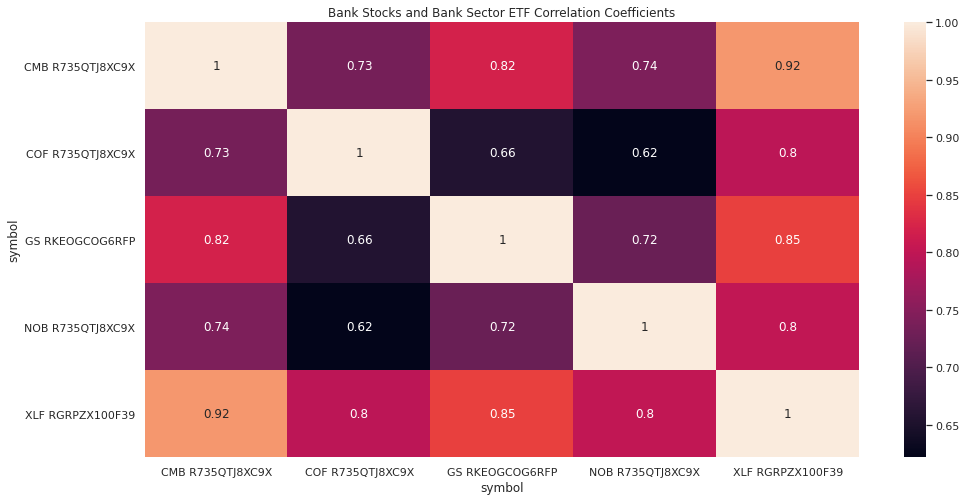### Create Pie Chart

You must import the plotting libraries and get some historical data to create pie charts.

In this example, you create a pie chart that shows the weights of the banking securities in a portfolio if you allocate to them based on their inverse volatility. Follow these steps to create the pie chart:

1. Select the close column and then call the unstack method.
2. close_prices = history['close'].unstack(level=0)
3. Call the pct_change method.
4. daily_returns = close_prices.pct_change()
5. Call var method and then take the inverse.
6. inverse_variance = 1 / daily_returns.var()
7. Call the color_palette method with a palette name and then truncate the returned colors to so that you have one color for each security.
8. colors = sns.color_palette('pastel')[:len(inverse_variance.index)]
9. Call the pie method with the security weights, labels, and colors.
10. plt.pie(inverse_variance, labels=inverse_variance.index, colors=colors, autopct='%1.1f%%')
11. In the same cell that you called the pie method, call the title method with a title.
12. plt.title(title='Banking Stocks and Bank Sector ETF Allocation');

The Jupyter Notebook displays the pie chart.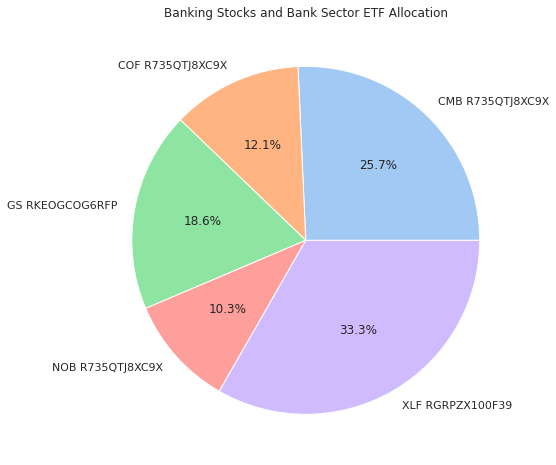You can also see our Videos. You can also get in touch with us via Discord.